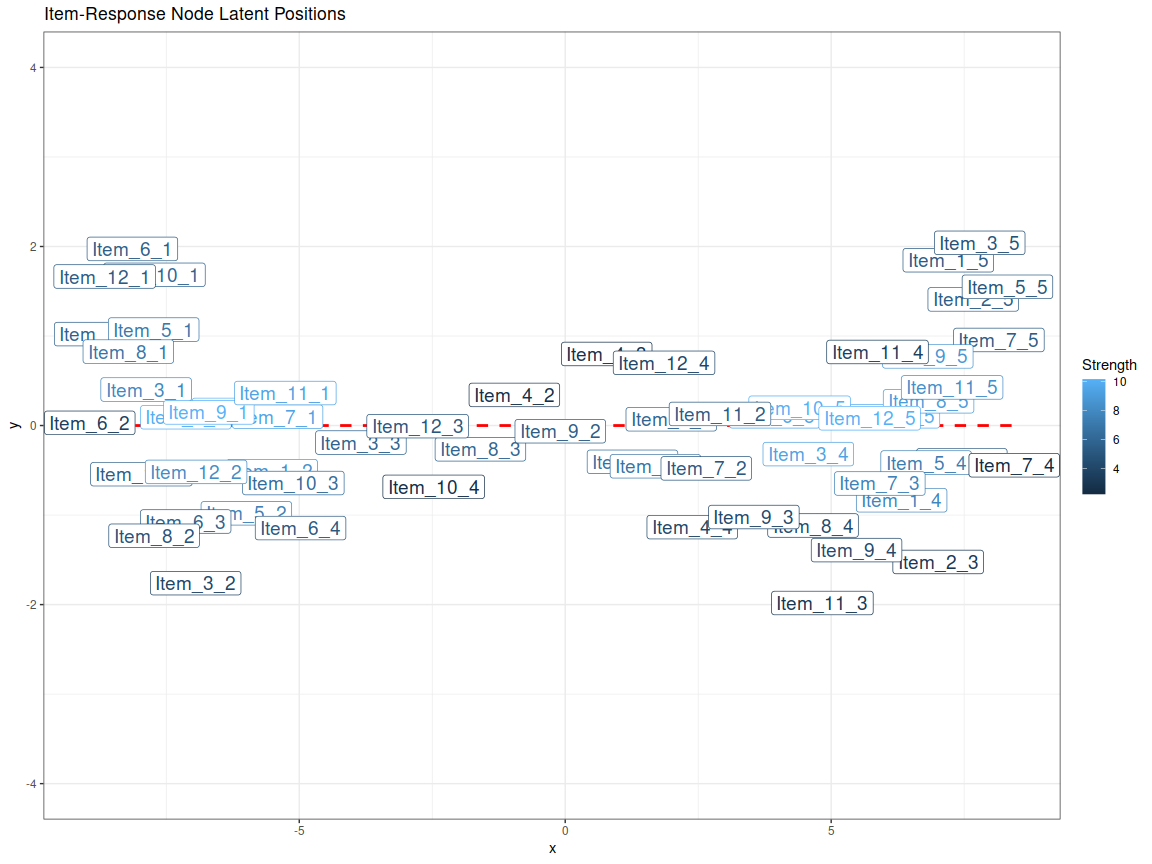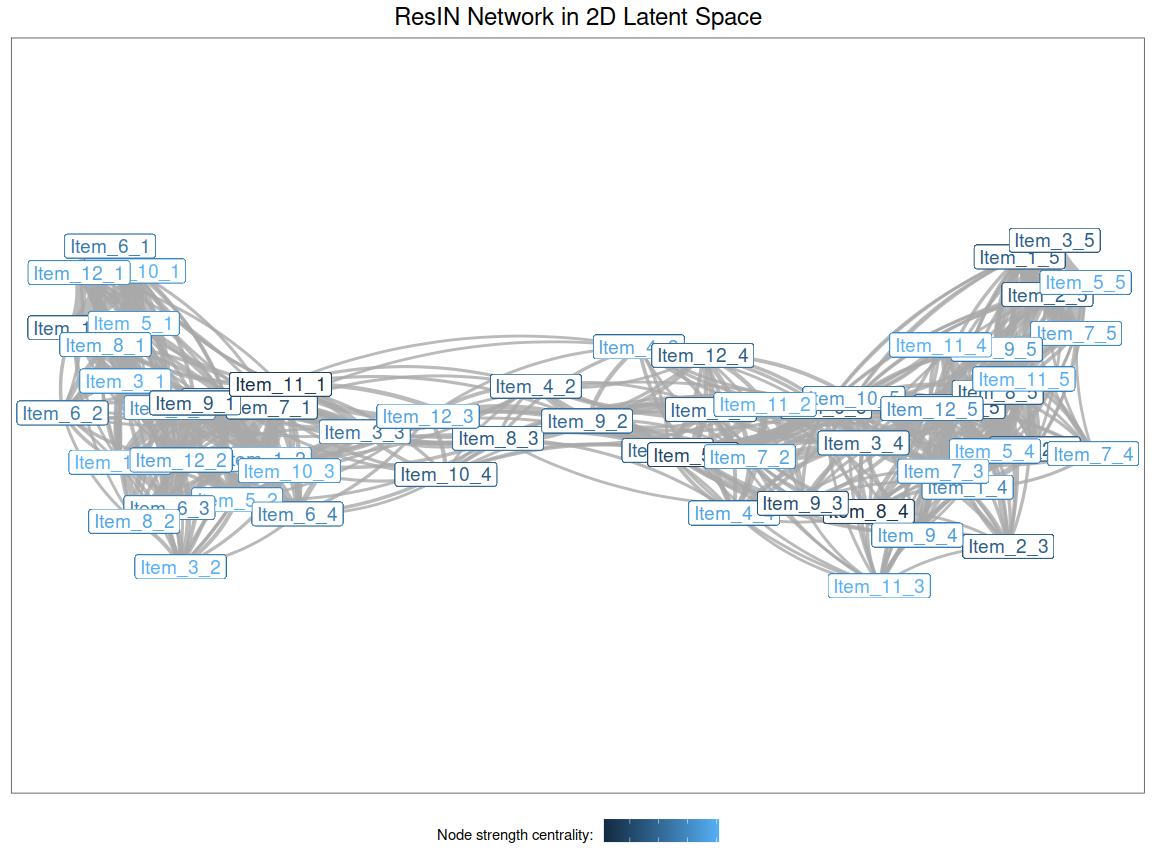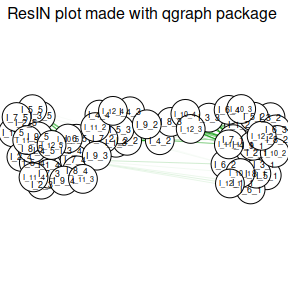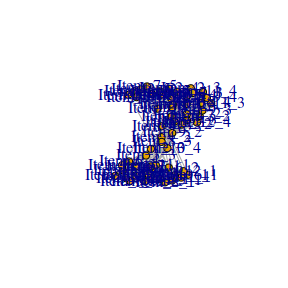# ResIN-VIGNETTE

# install.packages("ResIN")

library(ggplot2)
library(ResIN)

Welcome to the ResIN R-Package vignette. Here we’ll showcase and comment on some of the features available in the ResIN package.

## A simple example with simulated data:

We’ll first load a slightly noisy dataset of 8 Likert-scale items for 1000 hypothetical respondents:


data(lik_data)

### Running the ResIN algorithm

The first argument supplied to the function is simply the data-frame containing all (survey) items we would like to include in the analysis. Here, we also chose the correlation method that calculates the item-pair associations and specified that we’d like to estimate network descriptive statistics. All this can be done in one line of code.

# Apply the ResIN function to toy Likert data:

ResIN_output <- ResIN(lik_data, cor_method = "spearman", network_stats = TRUE)

### Visualize the network with ggplot:

The resulting object provides us both a node-list and an edge-list type data-frame. Let’s first use the node-list to locate each vertex in 2D space. We can simply rely on the x- and y-axis coordinates estimated by the force-directed algorithm and supply these as arguments to . While we’re at it, why not use a color aesthetic to visualize each node’s strength centrality. Finally, we are just re-scaling the y-axis to 50% of the x-axis to demonstrate that uni-dimensionality is a reasonable assumption for this attitude space:


ggplot(ResIN_output$node_frame, aes(x = x, y = y))+ geom_point(size = 0.25, color = "grey")+ geom_smooth(method = "lm", se = FALSE, linetype = 2, color = "red")+ geom_label(data = ResIN_output$node_frame, aes(x = x, y = y, label = node_names, color = Strength), size = 5)+
ylim(c(-4, 4))+
theme_bw()+
ggtitle("Item-Response Node Latent Positions")ResIN’s edge-list object provides us with much more detailed plotting (and analytic) capabilities. The amazing extension to lets us plot edges as a dedicated layer. We just need to make sure to plot node labels both based on the to and from columns in the plotting frame. Here, we scale the thickness of edges to the relative correlational strength between nodes.


edge_plot <- ggplot() +
geom_curve(data = ResIN_output$edgelist_frame, aes(x = from.x, xend = to.x, y = from.y, yend = to.y, size = weight^5), curvature = 0.15, color = "darkgrey", alpha = 0.8) + geom_label(data = ResIN_output$edgelist_frame, aes(x = from.x, y = from.y, label = from, color = Strength), size = 5) +
geom_label(data = ResIN_output$edgelist_frame, aes(x = to.x, y = to.y, label = to, color = Strength), size = 5) + ## Make sure to plot node labels both based on the to and from columns in the plotting frame ggtitle("ResIN Network in 2D Latent Space")+ labs(color = "Node strength centrality: ")+ ylim(c(-4, 4))+ scale_size_continuous(guide = "none")+ theme_bw()+ theme(axis.text.x = element_blank(), axis.title.x = element_blank(), axis.text.y = element_blank(), axis.title.y = element_blank(), axis.ticks = element_blank(), panel.grid.major = element_blank(), panel.grid.minor = element_blank(), legend.position = "bottom", legend.text = element_blank(), plot.title = element_text(hjust = 0.5, size=18)) edge_plot### qgraph and igraph integration: The ResIN package allows for simple qgraph and igraph integration for researchers who primarily use either of these packages for network analysis. Among other output, the and functions return S3 and -list objects, respectively. The and arguments conveniently pass a list of instructions to the underlying packages.  ## Integration with qgraph package resin_qgraph <- ResIN_qgraph(lik_data, plot_graph = TRUE, qgraph_arglist = list(layout = "spring", maximum = 1, vsize = 4, DoNotPlot = TRUE, sampleSize = nrow(lik_data), title = "ResIN plot made with qgraph package", mar = c(1,1,1,1), normalize = FALSE))## Integration with igraph package resin_igraph <- ResIN_igraph(lik_data) set.seed(12) igraph::plot.igraph(resin_igraph$igraph_obj)